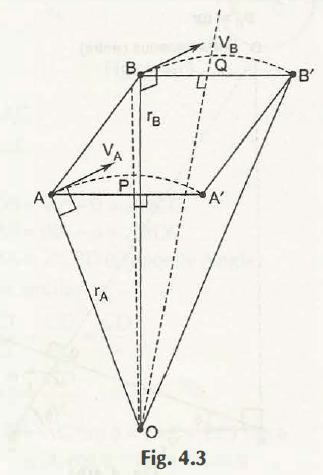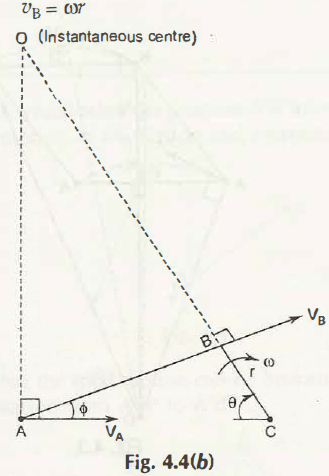Suppose the total motion of the link AB to reach A’B’ is entirely done by rotation about any point O. This point is known as the instantaneous centre of rotation.

To find such instantaneous centre of rotation, you have to follow certain steps-

• Draw perpendicular bisector of AA’ and BB’
• Mark it as PO and QO
• The point O is the instantaneous centre of rotationConsidering the Fig. 4.4 (b), it shows position of instantaneous centre.Let  be the angular velocity

VA = Linear velocity at point A = rA = AO

VB = Linear velocity at point B = BO

Hence,

VA/ VB = AO/ BO

Note:

Suppose we know the value of velocities at point A and B. The instantaneous centre can be obtained by perpendicular line drawing in the direction of these velocities.

Links of Next Mechanical Engineering Topics:-### Customer Reviews

My Homework Help
Rated 5.0 out of 5 based on 510 customer reviews at